# ExamplesHome Intro How They Work Characteristics EXAMPLES## Contents:

1. Torque/Speed Curves
2. 1.1 Examples From 2.007
3. 1.2 Multimedia Examples
4. Power Curves
5. 2.1 Example From 2.007

## Torque/Speed Curves

### Section 1.1 Examples from 2.007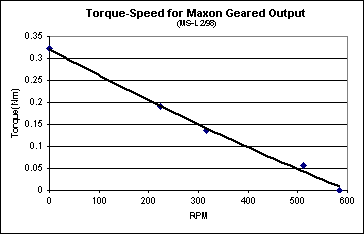The torque/speed curve to the right is for the green maxon geared D.C. motor used in a course at MIT: 2.007 Design and Manufacturing I.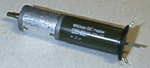(green maxon motor)

Note:
• The curve is for the output of the gearbox.
• The units given on the graph are N·m for torque, and rpm for rotational speed.
• no load speed,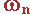= 600 rpm
• stall torque,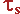= 0.325 N·m
• max power output, (Pmax= [31.416 rad/s * 0.163 N·m] = 5.105 W) occurs at= 300 rpm, and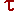= 0.163 N·m
• Units must be consistent throughout all calculations.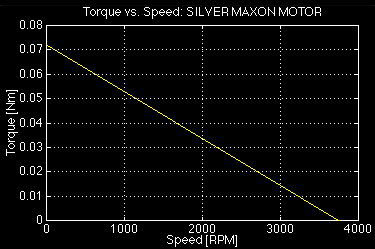The second torque/speed curve pictured here is for the silver maxon D.C. motor used in 2.007.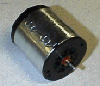(silver maxon motor)

Note:
• The curve is for the output shaft of the motor (it is not geared down).
• The units given on the graph are again N·m for torque, and rpm for rotational speed.
• no load speed,= 3750 rpm
• stall torque,= 0.0722 N·m
• max power output, (Pmax= [196.350 rad/s * 0.0361 N·m] = 7.088 W) occurs at= 1875 rpm, and= 0.0361 N·m
• Units must be consistent throughout all calculations.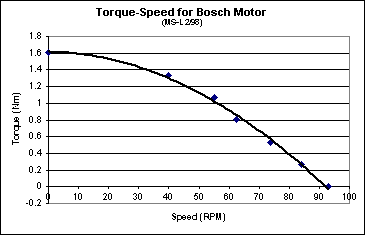This torque/speed curve is for the bosch D.C. wormgear motor used in 2.007.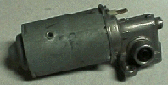(bosch wormgear motor)

Note:
• The curve is not linear due to the wormgear output
• The curve is for the wormgear output of the motor (the motor output shaft has a special wormgear pinion and carrier).
• The units given on the graph are again N·m for torque, and rpm for rotational speed.
• no load speed,= 92 rpm
• stall torque,= 1.6 N·m
• max power output, (Pmax= [4.82 rad/s * 0.80 N·m] = 3.85 W) still occurs at approximately= 46 rpm, and= 0.80 N·m
• Units must be consistent throughout all calculations.

Some helpful unit conversions are given below and in the Torque section of the Characteristics page.

### Section 1.2 Multimedia Examples

Here are some pictures and video clips of a clever device built here at MIT to illustrate how a torque/speed diagram represents the characteristics of an actual motor.

Be sure to listen to the motor as the video plays.
You can hear the motor strain as it is slowed down.

As the motor spins, the red weights are pulled further from the motor shaft by centrifugal force, which pulls the pen along the speed axis. As the experimenter slows the shaft down with her hand, the weights move in, pushing the pen to the left. Meanwhile, a torque is being applied to the motor shaft by the person's hand in order to slow it down. The motor is allowed to rotate, constrained by the spring attached to the plot area and the cable that wraps around the motor. From Newton's third law, we know that every action has an equal and opposite reaction, so the motor rotates as the torque is applied, pulling on the cable and moving the plot to draw torque on the vertical axis. Be sure to listen to the motor as it is slowed down. The motor's tendency to rotate as the shaft is slowed down is the same as that of an electric screwdriver as it rotates a screw. You have to hold the screwdriver firmly to keep it from spinning in your hand.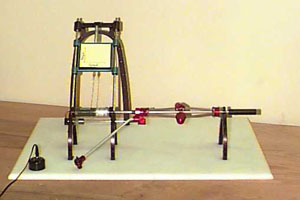Quicktime Video of the device as it is turned on, spins up to speed, is stalled out twice, and is tuned off.
(1.11 MB)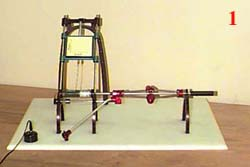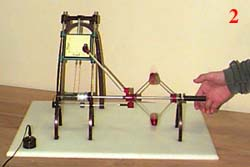1. At rest (no rotation). 2. At full speed (no load applied).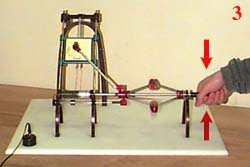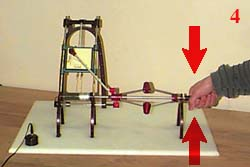3. Loaded to about half speed. 4. Stalled (maxium torque, no rotation).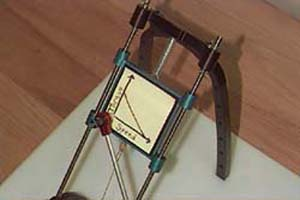This video is a close-up of the plot area as the curve is traced by the machine as above.
(0.744 MB)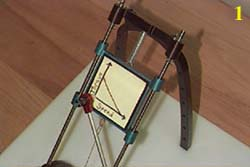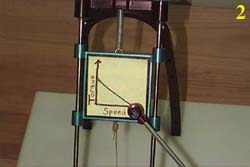1. At rest (no rotation). 2. At full speed (no load applied).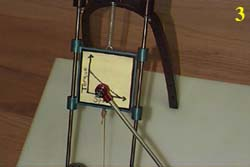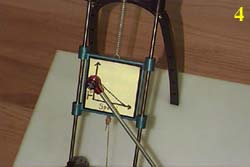3. Loaded to about half speed. 4. Stalled (maxium torque, no rotation).

## Power Curves

### Section 2.1 Example from 2.007

Below is the power curve for the green maxon motor pictured in the torque/speed section above. The yellow parabolic curve is a plot of Power vs. Speed, and the white linear curve is a plot of Power vs. Torque. Recall that power is the product of torque and rotational speed. As you can see from the plot, power is a maximum at the point on the torque/speed curve where torque and speed are equal to one half of their maximum values.* Note that the maximum power occurs at half the no-load speed and half the stall torque.

SI English
1 m = 100 cm
1 m = 1000 mm
1 cm = 10 mm
1 m = 3.280 ft
1 m = 39.370 in
1 cm = 0.394 in
1 N = 0.225 lbf
1 N = 3.597 oz
1 kN = 1000 N
1 ft = 12 in
1 in = 0.0833 ft

1 ft = 0.305 m
1 in = 2.540 cm

1 lbf = 4.448 N
1 oz = 0.278 N

1 W = 1 N·m/s
1 kW = 1000W>

1 W = 1.341E-03 hp
1 W = 0.738 ft·lb/s

1 kg = 2.205 lb*
1 g = 2.205E-03 lb*
1 g = 0.035 oz*
1 ft·lb/s = 1.818E-03 hp
1 ft·lb/s = 192 in·lb/s

1 ft·lb/s = 1.356 W
1 in·oz/s = 7.06E-03 W
1 hp = 745.70 W
1 lb = 16 oz
1 lb = 0.454 kg*
1 oz = 28.350 g*

* when the acceleration due to gravity, g = 9.80 m/s^2 (g = 32.15 ft/s^2)

For an extensive reference on units, check out this site: http://www.unc.edu/~rowlett/units/index.html

Home Intro How They Work Characteristics EXAMPLES

Webmaster: Matt Page mpage1@mit.edu